{{ message }}

# Bubble plot miscalculates size when all points within a color group are the same size#1133

Closed
opened this issue Oct 5, 2017 · 3 comments
Closed

# Bubble plot miscalculates size when all points within a color group are the same size#1133

opened this issue Oct 5, 2017 · 3 comments

###brianstamper commented Oct 5, 2017 • edited

 This bug can occur when creating a bubble plot where a discrete variable determines color and a continuous variable determines size. If all of the points within a given color are the same size (or if there is only one point within that group) then the bubbles are sized incorrectly. When using `sizemode = 'diameter'` this issue does not occur, it only happens with the default `sizemode = 'area'`. Based on the experiments shown below it appears that these problematic points are having the size computed as diameter instead of area in either case, as they appear to have the same size in both modes. I first asked about this on SO at (https://stackoverflow.com/questions/46571892/r-plotly-scatter-plot-marker-size-appears-to-depend-on-color-when-color-is-discr) I did not include examples where color is based on a numeric or integer instead of factor or character, but I can attest this bug does not occur in those cases. (In cases where the legend is a gradient instead of discrete values.) Possibly related to #755 about size of bubbles in the legend? ```library(plotly) # In these plots I will set x, y, and size to the same variable, so the # largest bubbles should appear on the top-right and smallest on the # bottom-left. The bug still occurs if you set x and y to other variables, # but it becomes less obvious to see the phenomenon being described. # Make a version of mtcars where 'carb' is a discrete variable. Here I # make it a factor, but as.character() also works to demonstrate this bug. my_mtcars <- mtcars my_mtcars\$carb <- as.factor(my_mtcars\$carb) # With the default sizemode = 'area', we have the bug, for the # points corresponding to my_mtcars\$carb %in% c(6, 8) plot_ly(my_mtcars, x = ~wt, y = ~wt, size = ~wt, color = ~carb, type = 'scatter', mode = 'markers', marker = list(sizemode = 'area')) # This is actually the default```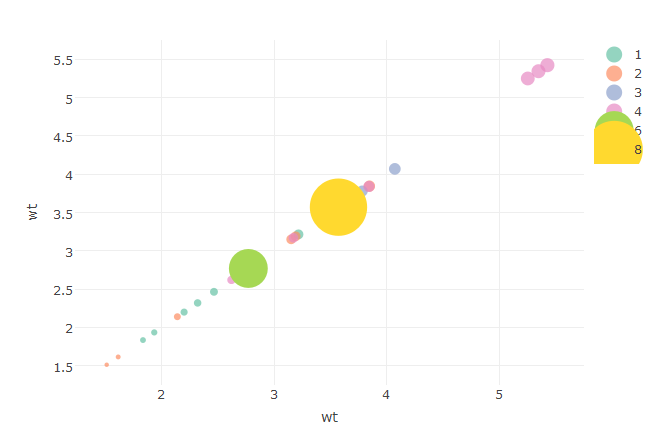```# Set sizemode = 'diameter' and the bug goes away # (though I notice the problematic bubbles are the same size as # they were in the plot with the sizemode = 'area'... hmm...) plot_ly(my_mtcars, x = ~wt, y = ~wt, size = ~wt, color = ~carb, type = 'scatter', mode = 'markers', marker = list(sizemode = 'diameter'))```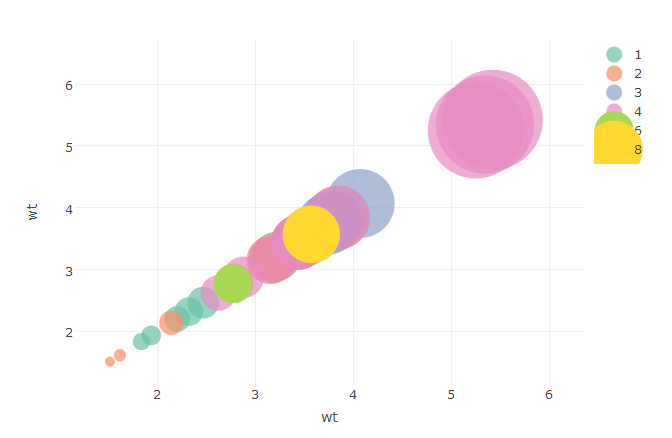```# If the points in each color group have distinct sizes, then the bug doesn't occur my_iris <- iris plot_ly(my_iris, x = ~Sepal.Length, y = ~Sepal.Length, size = ~Sepal.Length, color = ~Species, type = 'scatter', mode = 'markers', marker = list(sizemode = 'area'))```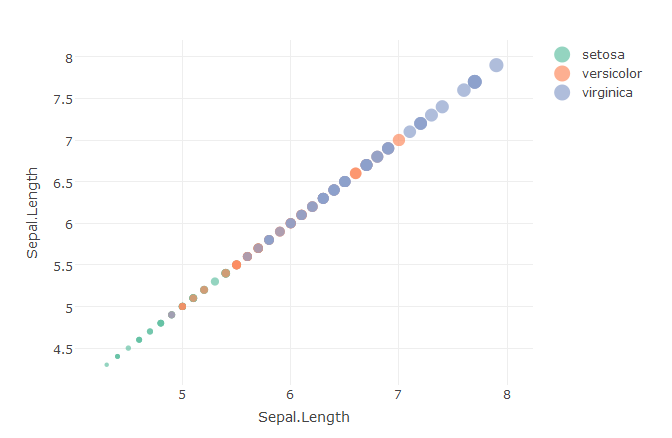```# But if all of the points in a group are the same size, then the bug appears my_iris[my_iris\$Species == 'virginica', ]\$Sepal.Length <- median(my_iris\$Sepal.Length) plot_ly(my_iris, x = ~Sepal.Length, y = ~Sepal.Length, size = ~Sepal.Length, color = ~Species, type = 'scatter', mode = 'markers', marker = list(sizemode = 'area'))```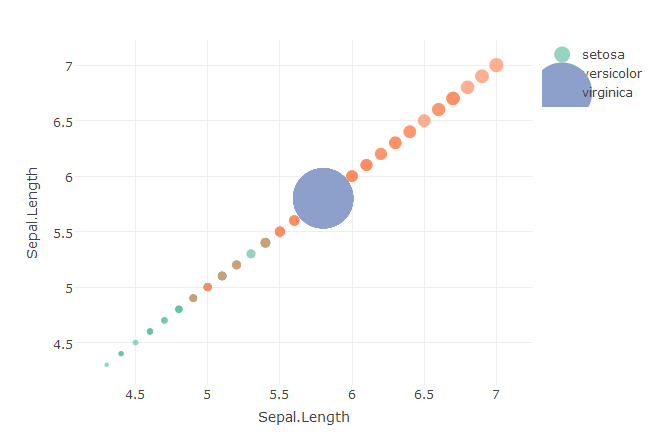```# Put a little jiggle on that Sepal.Length and the bug goes away again my_iris\$Sepal.Length <- my_iris\$Sepal.Length + rnorm(nrow(my_iris), sd = .0001) plot_ly(my_iris, x = ~Sepal.Length, y = ~Sepal.Length, size = ~Sepal.Length, color = ~Species, type = 'scatter', mode = 'markers', marker = list(sizemode = 'area'))```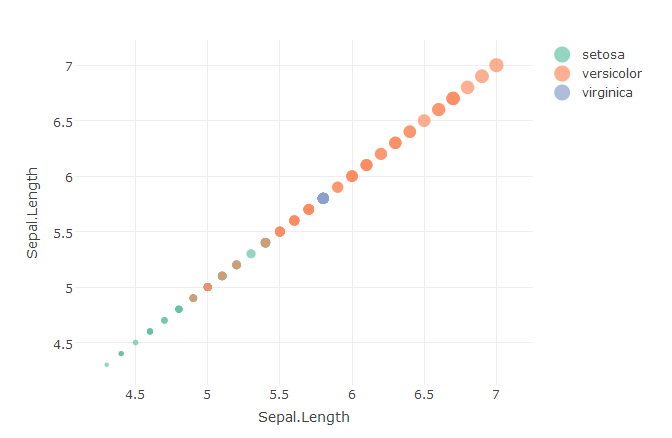The text was updated successfully, but these errors were encountered:
We are unable to convert the task to an issue at this time. Please try again.

###micdonato commented Jun 13, 2018 • edited

 Oh wow, I thought I was the only one having this issue. Thanks for your examples, it helped me solved it! I tried to post about it on the plotly community forum, but nobody replied... Also, great find on the added noise!

###cpsievert commented Jun 13, 2018 • edited

 Sorry for the late response and thanks for the thorough report! Note how those problematic traces (e.g., carb of 6 or 8) have just one data point. For those traces, `marker.size` is currently translated to a number rather than an array of length one. Unfortunately, plotly.js currently ignores `marker.sizemode` when `marker.size` is a number. Maybe someday this will fixed in plotly.js itself, but I think the right way to proceed is to make sure the R package always translates `marker.size` to an array when `marker.sizemode="area"`

###brianstamper commented Jun 14, 2018

 Thanks for the update. A minor (probably quibbling) point - I don't think having just one data point in a group is a requirement for this to occur, for example in the second `iris` example above I made many points in a group share the same value. Perhaps this doesn't matter because when markers are constructed non-distinct values are discarded, leading to this number vs. array of length one issue? Admittedly I'm guessing, I haven't tried installing the development version with this patch to test it out.